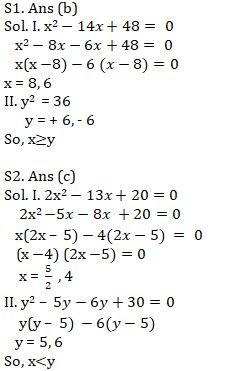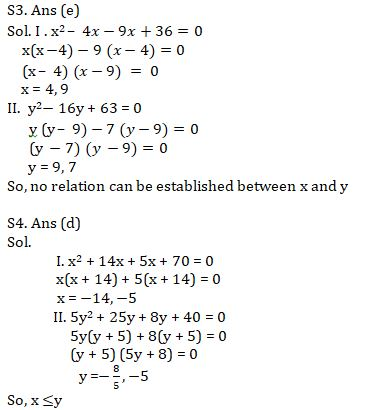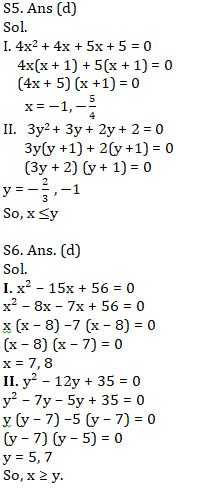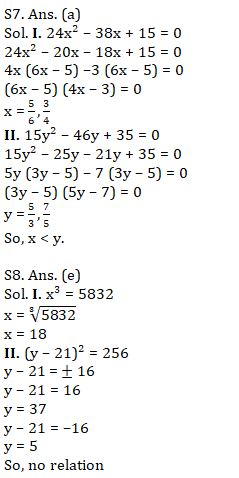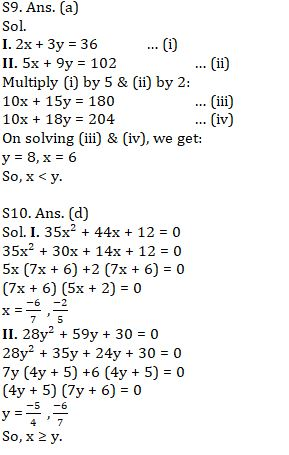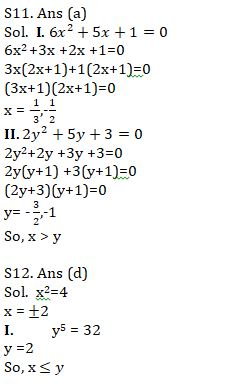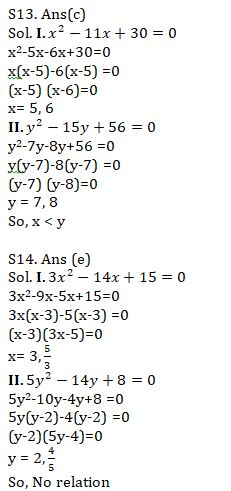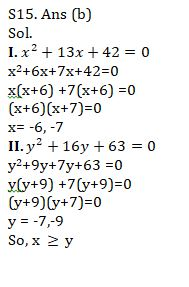Latest Banking jobs   »   Quantitative Aptitude Quiz For IBPS Clerk...

# Quantitative Aptitude Quiz For IBPS Clerk Prelims 2021- 5th August

Direction (1 – 5): Solve the given quadratic equations and mark the correct option based on your answer.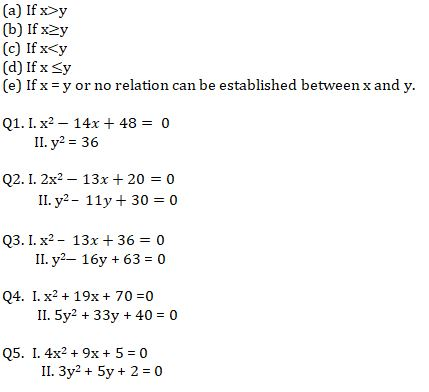Directions (6-10): In the following questions, two equations (I) and (II) are given. You have to solve both the equations and mark the appropriate answer.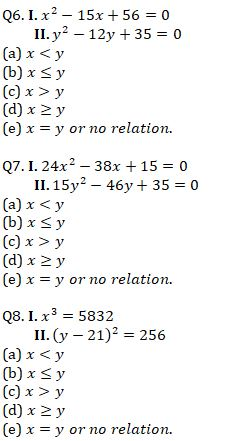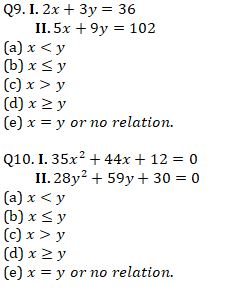Directions (11-15):- In each of the following questions, two equations (I) and (II) are given. Solve the equations and mark the correct option: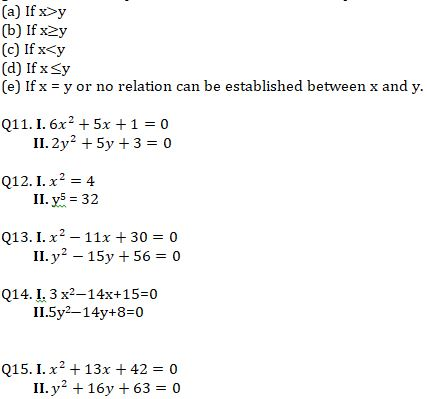Solution